# Concave lens – Object at different distances from the lens

When viewing an object through a convex lens, the observer will always see an upright, smaller version, for all positions of the object.

This can be demonstrated as follows.

## Object at more than 2F

This means that the object is further away than two principal focal lengths from the concave lens.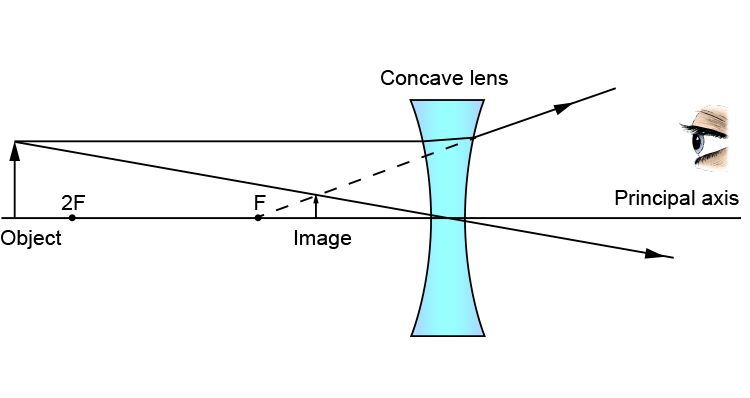The image is smaller, upright, virtual and between the principal focal point on the object side and the concave lens.

## Object at 2F

When the object is at two principal focal points from the concave lens, you get the following ray diagram: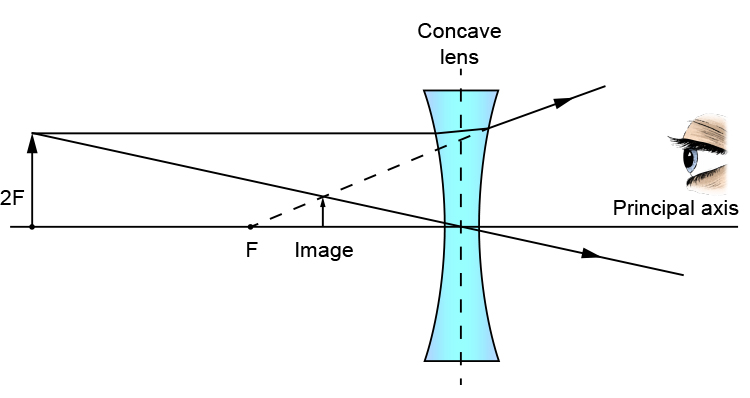The image is smaller, upright, virtual and between the principal focal point on the object side and concave lens.

## Object between 2F and F

Placing the object between two focal points and one focal point produces the following ray diagram: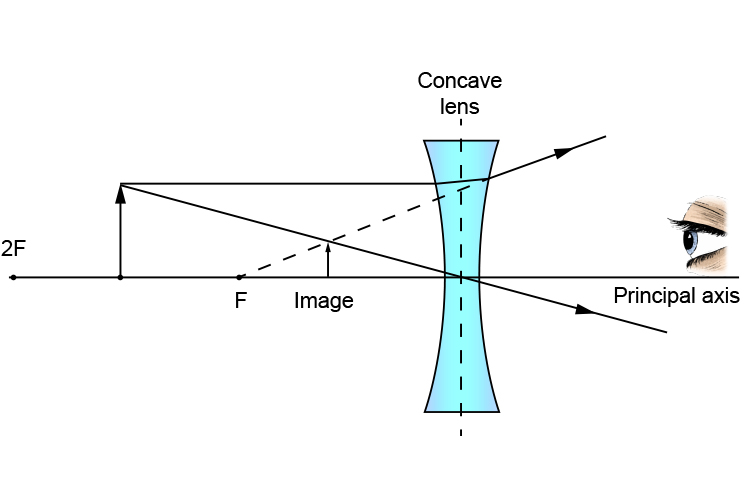The image is smaller, upright, virtual and between the principal focal point on the object side and the concave lens.

## Object at F

When the object is placed at the focal point of the concave lens, you get the following ray diagram: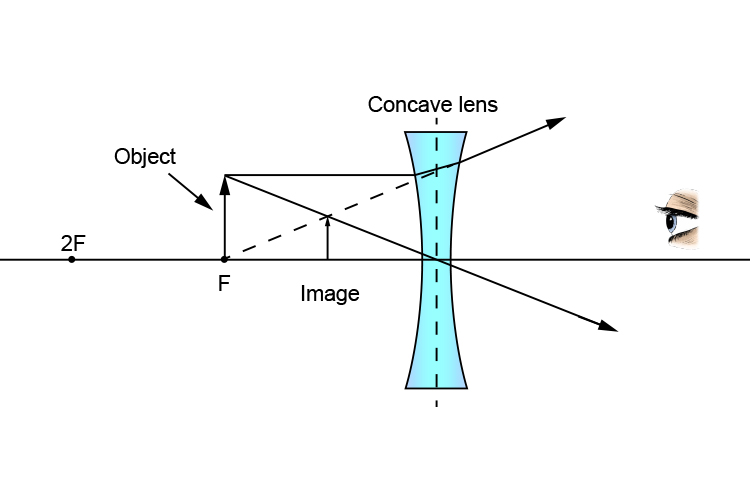The image is smaller, upright, virtual and between the principal focal point on the object side and the concave lens.

## Object between F and concave lens

When the object is placed between the principal focal point and the lens, the following occurs: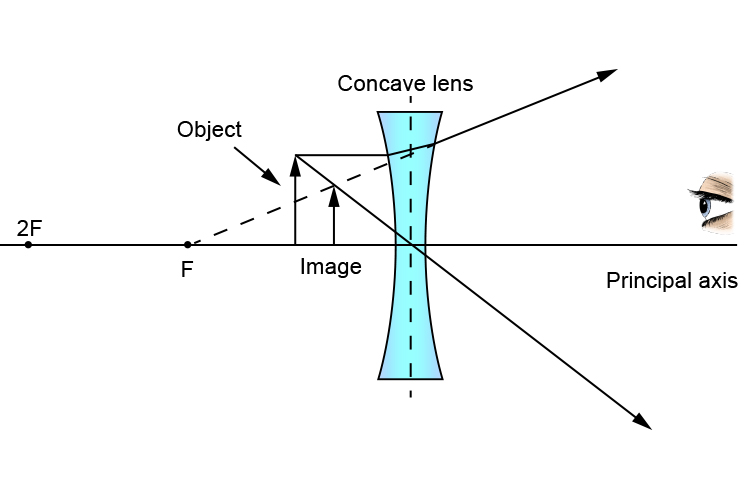The image is smaller, upright, virtual and between the principal focal point on the object side and the concave lens.

## Conclusion

We can see from the above that for every position of the object the image created is always.

SMALLER

UPRIGHT

VIRTUAL (can not be projected on a screen)

Between principal focal point and the concave lens

All images through a concave lens will look smaller and closer.